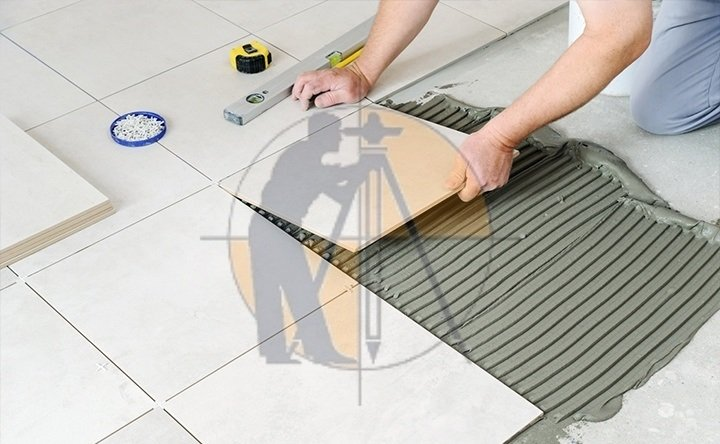Breaking News
Home / Civil Engineering / How to calculate the Number of tiles

# How to calculate the Number of tiles

### What are the tiles?

This is the thin and rectangular shape of material. This is used for floor wall and road etc.### What is the purpose of tiles?

The used of tiles for different purpose some area are used for covering and some area used for good looking. Different types are used in construction as like ceramic tiles gray night tiles limestone cement tiles and glass tile etc.

### Given data

Length of room =14ft

Width of room =12ft

Length of one tile=2ft

Width of one tile =1ft

### Now

Area of room =L x W

### Area of room  = 14 x 12 =168ft2

Area of one tile =L x W

### Area of one tile =2×1=2ft2

Now we know the area of room and the area of one tile

Number of tiles= area of room/area of one tile

## . Land Surveying & Architects

### THANKS.